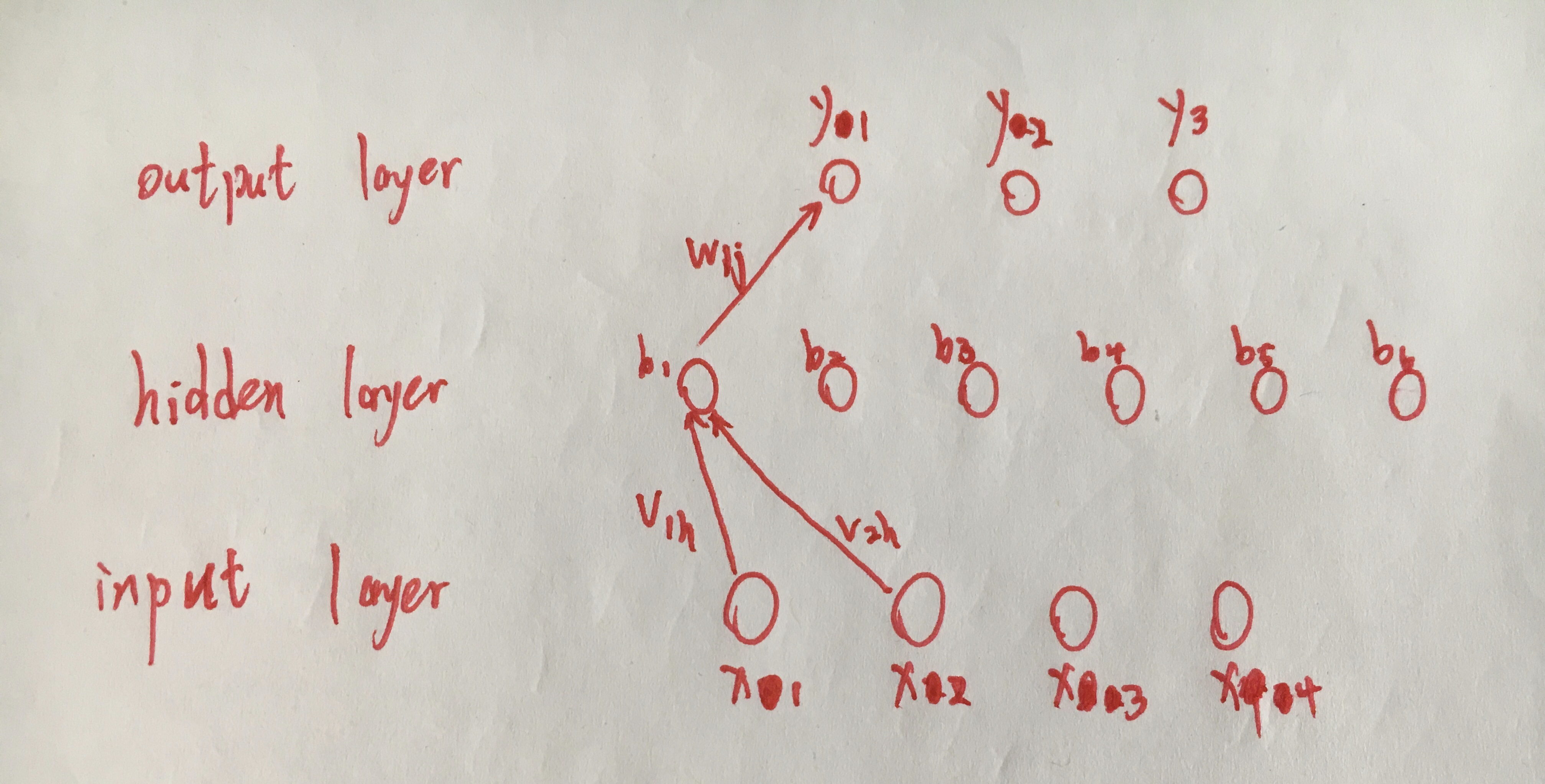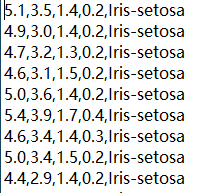# 【模式识别小作业】单隐层神经网络（neural network)+Matlab实现+UCI的Iris和Seeds数据集+分类问题

## 1.Inroduction

In this assignment, I implemented the predictive modeling approach based on the neural network to do the three-classification tasks on two data sets by using MATLAB. The data sets, Iris and Seeds, are downloaded from the UCI Machine Learning Repository.

Each program contains one .m file, which is processed to estimate the values of all weight and threshold based on the test data set. Taking the data set Iris as an example, the entire neural network is designed as shown below.At the input layer, there are four features because the data set contains four features. Six hidden nodes are embedded at the hidden layer, considering the speed of operation and the amount of data. Since there are three types of flowers in the dataset, three nodes are set at the output layer.

Experimental results show that using the neural network model on Iris data set to solve the three-classification problems looks good. Because, I think, the number of the sample and attribute of Iris is little, the processing speed is fast and accuracy is high. After matching all weight and threshold of the nodes on the train data set, the samples on the test data set can be classified correctly by this network. While the effect on Seeds data set is not good. The data set contains 7 features. I think that using the neural network of only one hidden layer to train the model will not perform well. It may be necessary to perform some data dimensionality reduction operations before training the model.

## 2.The characteristics of the data sets

2.1 Iris
The data set contains 150 samples. Every sample have 4 attributes: sepal length in cm, sepal width in cm, petal length in cm, and petal width in cm. Three classes of Iris is Iris Setosa, Iris Versicolour and Iris Virginica. This data set is the most popular in UCI. The clear structure and plentiful samples make it suitable in this assignment.

2.2 Seeds
The data set contains 210 samples. Every sample have 7 attributes: area A, perimeter P, compactness C = 4piA/P^2, length of kernel, width of kernel, asymmetry coefficient and length of kernel groove. And all of these parameters were real-valued continuous. The data set comprises kernels belonging to three different varieties of wheat: Kama, Rosa and Canadian. It is often used for the tasks of classification and cluster analysis.

## 3.Data preprocessing

Taking the Iris as an example, the first step is to convert characters in data set into digital representations.Read the dataset file with MATLAB, extract the whole attributes into an array, and merge the numeric symbols after each sample. Iris-setosa is marked as 1, Iris-versicolor is marked as 2, and Iris-virginica is marked as 3. Then, the hold-out method is used to extract one out of every 5 samples to form a test set. The remaining 80 samples are used as the training set. And write these data set to each txt file to facilitate saving and using.

## 4.The code

%This program is based on the data set Iris
%The original data set contains three kinds of flowers
%Here, the neuralnetword method is processed to do the three-category problem
%The flower name in the data set is changed to a numeric symbol of 1 2 3

clear;
clc;

%%%%%%%%%%%%%%Data preprocessing section%%%%%%%%%%%%%%%%
f=fopen('iris.data');%Open dataset file
data=textscan(f,'%f,%f,%f,%f,%s'); %Read file content

D=[];% Used to store attribute values
for i=1:length(data)-1
D=[D data{1,i}];
end
fclose(f);

lable=data{1,length(data)};
n1=0;n2=0;n3=0;
% Find the index of each type of data
for j=1:length(lable)
if strcmp(lable{j,1},'Iris-setosa')
n1=n1+1;
index_1(n1)=j;% Record the index belonging to the "Iris-setosa" class

elseif strcmp(lable{j,1},'Iris-versicolor')
n2=n2+1;
index_2(n2)=j;% Record the index belonging to the "Iris-versicolor" class

elseif strcmp(lable{j,1},'Iris-virginica')
n3=n3+1;
index_3(n3)=j;% Record the index belonging to the "Iris-virginica" class

end
end

% Retrieve each type of data according to the index
class_1=D(index_1,:);
class_2=D(index_2,:);
class_3=D(index_3,:);
Attributes=[class_1;class_2;class_3];

%Iris-setosa is marked as 0; Iris-versicolor is marked as 1
%Iris-virginica is marked as 2
I=[1*ones(n1,1);2*ones(n2,1);3*ones(n3,1)];
Iris=[Attributes I];% Change the name of the flower to a number tag

save Iris.mat Iris % Save all data as a mat file

%Save all data as a txt file
f=fopen('iris1.txt','w');
[m,n]=size(Iris);
for i=1:m
for j=1:n
if j==n
fprintf(f,'%g \n',Iris(i,j));
else
fprintf(f,'%g,',Iris(i,j));
end
end
end
fclose(f);

%Use the set-out method to extract one out of every 5 data, a total of 30 data to form a test set
f_test=fopen('iris_test.txt','w');
[m,n]=size(Iris);
for i=1:m
if rem(i,5)==0
for j=1:n
if j==n
fprintf(f_test,'%g \n',Iris(i,j));
else
fprintf(f_test,'%g,',Iris(i,j));
end
end
end
end
fclose(f_test);

%The remaining 120 data as a training set
f_train=fopen('iris_train.txt','w');
[m,n]=size(Iris);
for i=1:m
if rem(i,5) ~=0
for j=1:n
if j==n
fprintf(f_train,'%g \n',Iris(i,j));
else
fprintf(f_train,'%g,',Iris(i,j));
end
end
end
end
fclose(f_train);

%%%%%%%%%%%%%%%%%%Initialize all connection rights and thresholds%%%%%%%
l=3; %Number of categories
q=6; %Number of nodes
d=4; %Number of features

anta=0.1; %Learning rate

v=rand(d,q);
b_cita=rand(1,q);
w=rand(q,l);
y_cita=rand(1,l);

%Save initialization results
initialization=fopen('initialization.txt','w');
[m,n]=size(v);
for i=1:m
if i==1
fprintf(initialization,'v: \n');
end
for j=1:n
if j==n
fprintf(initialization,'%g \n',v(i,j));
else
fprintf(initialization,'%g,',v(i,j));
end
end
if i==m
fprintf(initialization,' \n');
end
end
[m,n]=size(b_cita);
for i=1:m
if i==1
fprintf(initialization,'b_cita: \n');
end
for j=1:n
if j==n
fprintf(initialization,'%g \n',b_cita(i,j));
else
fprintf(initialization,'%g,',b_cita(i,j));
end
end
if i==m
fprintf(initialization,' \n');
end
end
[m,n]=size(w);
for i=1:m
if i==1
fprintf(initialization,'w: \n');
end
for j=1:n
if j==n
fprintf(initialization,'%g \n',w(i,j));
else
fprintf(initialization,'%g,',w(i,j));
end
end
if i==m
fprintf(initialization,' \n');
end
end
[m,n]=size(y_cita);
for i=1:m
if i==1
fprintf(initialization,'y_cita: \n');
end
for j=1:n
if j==n
fprintf(initialization,'%g \n',y_cita(i,j));
else
fprintf(initialization,'%g,',y_cita(i,j));
end
end
if i==m
fprintf(initialization,' \n');
end
end
fclose(initialization);
%%%%%%%%%%%%%%%%%%%%%%%%%%%%%%%%%%%%%%%%%%%%%%%%%%%%%%%%%%%%%%%%%%%%%

y_lab=iris_train(:,5); %Read the floral label in the fifth column
x=iris_train(:,1:4); %Read four attribute values
y_lab_test=iris_test(:,5); %Read the floral label in the fifth column
x_test=iris_test(:,1:4); %Read four attribute values

%Calculate the number of samples for training and test samples
[yangbenshu,tezhengshu]=size(iris_train);
[yangbenshu_test,tezhengshu_test]=size(iris_test);

diedai=1; %Number of iterations

%Used to save the calculated cumulative error
%the first element is the last calculation
%the second is the latest calculation
Etrain_ave=[0;0];
Etest_ave=[9999;0];

yout_train=zeros(yangbenshu,l); %Output the final y value of each sample
yout_test=zeros(yangbenshu_test,l);

%main
while 1

E=zeros(yangbenshu,1); %Initialization error variable
E_test=zeros(yangbenshu_test,1);

%Traversing the test set to update weights and thresholds
for yangben=1:yangbenshu

%The label is 1, the vector of y is 1,0,0
%The label is 2, the vector of y is 0,1,0
%The label is 3, the vector of y is 0,0,1
if y_lab(yangben)==1
y_true(1)=1;y_true(2)=0;y_true(3)=0;
end
if y_lab(yangben)==2
y_true(1)=0;y_true(2)=1;y_true(3)=0;
end
if y_lab(yangben)==3
y_true(1)=0;y_true(2)=0;y_true(3)=1;
end

%%%%%%%%%A complete calculation process%%%%%%
for m=1:q
afang(m)=0;
end
for m=1:l
baita(m)=0;
end

for h=1:q
for i=1:d
afang(h)=afang(h)+v(i,h)*x(yangben,i);
end
end

b_qian=afang-b_cita;

for h=1:q
b(h)=1/(1+exp((-1)*b_qian(h)));
end

for j=1:l
for h=1:q
baita(j)=baita(j)+w(h,j)*b(h);
end
end

y_qian=baita-y_cita;

for j=1:l
y(j)=1/(1+exp((-1)*y_qian(j)));
yout_train(yangben,j)=y(j);
end
%%%%%%%%%%%%%%%%%%%%%%%%%%%%%%%%%%%%%%%%%%%%%

%%%%%%%%%%%%%%%BP algorithm%%%%%%%%%%%%%%%%%%
%Mean square error calculation
for j=1:l
E(yangben)=E(yangben)+(1/2)*(y(j)-y_true(j))*(y(j)-y_true(j));
end

for j=1:l
g(j)=y(j)*(1-y(j))*(y_true(j)-y(j));
end

summ=0;
for h=1:q
for j=1:l
summ=summ+w(h,j)*g(j);
end
e(h)=b(h)*(1-b(h))*summ;
end

for h=1:q
for j=1:l
dta_w(h,j)=anta*g(j)*b(h);
end
end

for j=1:l
dta_ycita(j)=(-1)*anta*g(j);
end

for i=1:d
for h=1:q
dta_v(i,h)=anta*e(h)*x(yangben,i);
end
end

for h=1:q
dta_bcita(h)=(-1)*anta*e(h);
end

%update data
v=v+dta_v;
b_cita=b_cita+dta_bcita;
w=w+dta_w;
y_cita=y_cita+dta_ycita;

%%%%%%%%%%%%%%%%%%%%%%%%%%%%%%%%%%%%%%%%%%%%%%%%

end

%Calculate the cumulative error on the training set
for yangben=1:yangbenshu
Etrain_ave(2)=Etrain_ave(2)+(1/yangbenshu)*E(yangben);
end

%Calculate the mean square error on the test set
for yangben=1:yangbenshu_test

if y_lab_test(yangben)==1
y_true_test(1)=1;y_true_test(2)=0;y_true_test(3)=0;
end
if y_lab_test(yangben)==2
y_true_test(1)=0;y_true_test(2)=1;y_true_test(3)=0;
end
if y_lab_test(yangben)==3
y_true_test(1)=0;y_true_test(2)=0;y_true_test(3)=1;
end

for m=1:q
afang_test(m)=0;
end
for m=1:l
baita_test(m)=0;
end

for h=1:q
for i=1:d
afang_test(h)=afang_test(h)+v(i,h)*x_test(yangben,i);
end
end

b_qian_test=afang_test-b_cita;

for h=1:q
b_test(h)=1/(1+exp((-1)*b_qian_test(h)));
end

for j=1:l
for h=1:q
baita_test(j)=baita_test(j)+w(h,j)*b_test(h);
end
end

y_qian_test=baita_test-y_cita;

for j=1:l
y_test(j)=1/(1+exp((-1)*y_qian_test(j)));
yout_test(yangben,j)=y_test(j);
end

for j=1:l
E_test(yangben)=E_test(yangben)+(1/2)*(y_test(j)-y_true_test(j))*(y_test(j)-y_true_test(j));
end
end

%Calculate the cumulative error on the test set
for yangben=1:yangbenshu_test
Etest_ave(2)=Etest_ave(2)+(1/yangbenshu_test)*E_test(yangben);
end

%Using early stop method to alleviate over-fitting of BP network
if (Etrain_ave(2)<=Etrain_ave(1))&&(Etest_ave(2)>=Etest_ave(1))&&(diedai>500)
break;
end

%Numerical transfer
Etrain_ave(1)=Etrain_ave(2);
Etrain_ave(2)=0;
Etest_ave(1)=Etest_ave(2);
Etest_ave(2)=0;

%Calculate the iterations
diedai=diedai+1;
end

%Save the calculation result of the last weight and threshold
result=fopen('result.txt','w');
[m,n]=size(v);
for i=1:m
if i==1
fprintf(result,'v: \n');
end
for j=1:n
if j==n
fprintf(result,'%g \n',v(i,j));
else
fprintf(result,'%g,',v(i,j));
end
end
if i==m
fprintf(result,' \n');
end
end
[m,n]=size(b_cita);
for i=1:m
if i==1
fprintf(result,'b_cita: \n');
end
for j=1:n
if j==n
fprintf(result,'%g \n',b_cita(i,j));
else
fprintf(result,'%g,',b_cita(i,j));
end
end
if i==m
fprintf(result,' \n');
end
end
[m,n]=size(w);
for i=1:m
if i==1
fprintf(result,'w: \n');
end
for j=1:n
if j==n
fprintf(result,'%g \n',w(i,j));
else
fprintf(result,'%g,',w(i,j));
end
end
if i==m
fprintf(result,' \n');
end
end
[m,n]=size(y_cita);
for i=1:m
if i==1
fprintf(result,'y_cita: \n');
end
for j=1:n
if j==n
fprintf(result,'%g \n',y_cita(i,j));
else
fprintf(result,'%g,',y_cita(i,j));
end
end
if i==m
fprintf(result,' \n');
end
end
fclose(result);

%Save the y value of each sample in the training set
r_train=fopen('yout_train.txt','w');
[m,n]=size(yout_train);
for i=1:m
for j=1:n
if j==n
fprintf(r_train,'%g \n',yout_train(i,j));
else
fprintf(r_train,'%g,',yout_train(i,j));
end
end
end
fclose(r_train);

%Save the y value of each sample in the test set
r_test=fopen('yout_test.txt','w');
[m,n]=size(yout_test);
for i=1:m
for j=1:n
if j==n
fprintf(r_test,'%g \n',yout_test(i,j));
else
fprintf(r_test,'%g,',yout_test(i,j));
end
end
end
fclose(r_test);



## 5.Limitations and improvements

1. This neural network model can be applied to the second data set Seeds, but the effect is not good. It deserves improving further, perhaps by increasing the number of the hidden layers and nodes.
2. The principle and algorithm of the decision tree are not particularly clear for me, and further familiarity are needed.
3. Only three-classification tasks are considered in this assignment, and application of the neural network model to the multi-classification tasks and multi-layer network should be tried in the future.

### 评论

###### Your browser is out-of-date!

Update your browser to view this website correctly. Update my browser now

×